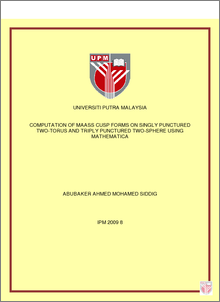# Computation of Maass Cusp Forms on Singly Punctured Two-Torus and Triply Punctured Two-Sphere Using Mathematica

## Citation

Mohamed Siddig, Abubaker Ahmed (2009) Computation of Maass Cusp Forms on Singly Punctured Two-Torus and Triply Punctured Two-Sphere Using Mathematica. PhD thesis, Universiti Putra Malaysia.

## Abstract

The topic of this study is the computation of Maass cusp form, i.e. the eigenfunctions of the hyperbolic Laplace-Beltrami operator on punctured surfaces namely singly punctured two-torus and triply punctured two-sphere. Punctured surfaces are surfaces with points removed or located infinitely far away and they have complex topological and geometrical properties. The presence of the punctures or cusps means that there is a continuous spectrum as well as the discrete one. This work focuses on the discrete part of computational nature. Hejhal developed an algorithm to compute Maass cusp form on triangle groups. The algorithm of Hejhal is based on automorphy condition and also applies to the computation of the Maass cusp forms on Fuchsian group whose the fundamental domain has exactly one cusp. In this work the method due to Hejhal was recalled and extended for computation of Maass cusp on singly punctured two-torus which still has one cusp but a nonzero genus. The algorithm was modified further to carry out the computation for the surfaces with three cusps i.e. triply punctured two-sphere. All the computations were implemented in Mathematica and built in a way accessible to any one with an introductory knowledge in Mathematica. The results of the study are the first low-lying eigenvalues, examples of Fourier coefficients and graphic plots of Maass cusp forms each for modular group, singly punctured two-torus and triply punctured two-sphere. The eigenvalues and the Fourier coefficients were computed with the desired accuracy. Some comparisons between singly punctured two-torus and triply punctured two-sphere are also presented.Preview
PDF
ABS_---__IPM_2009_8.pdfView Item